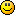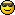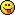## Discuss Scratch

i think that scratch should have an
`(i ::: operators)`
block
(i is the square root of -1.)

I think it would be too complex. So, no support.

R4356th wrote:

I think it would be too complex. So, no support.
is that a pun?
because numbers of the form a+bi are called complex numbersOut of curiosity — and this is for someone else to answer, not me — how often are complex numbers used in programming? I don't know of many uses for this other than maybe a complex number calculator.

Complex numbers are a little tricky, though. Workarounds do exist, although perhaps not very “clean” ones. Would you expect other blocks to adapt to complex numbers, then? For instance, would

`((i) * (i)) // colored orange for visibility`

return –1? And would

`([sqrt v] of (-1))`

return i? The issue with this one is that it might convince elementary school students that you can take square roots of negative numbers — which is true, but in a grade-school standpoint, students are not expected to know this yet.

Moreover, this would especially be troublesome if you try to do

`([sqrt v] of (i))`

as there are two square roots of i. Namely, (1+i)/sqrt(2) and (–1–i)/sqrt(2). I suppose you could make it return the principal root?

Furthermore, complex exponentials require some thought, as well. We'd need to make sure that

`([e^ v] of ((i) * (3.14159)) :: operators)`

returns something close to 1, and similarly for other complex exponentials. Complex trigonometry is also tricky; would we expect

`([arccos v] of (2) :: operators)`

to give us an answer? Ordinarily, no — but there is a complex number z such that cos(z) = 2, so this block should return something in terms of complex numbers.

Complex analysis is very tricky indeed. I feel like it could create unexpected issues in some pieces of code. But I imagine it could work. It all depends on, however, whether this really proves to be useful for Scratch programming.

This is my forum signature! On a forum post, it is okay for Scratchers to advertise in their forum signature. The signature is the stuff that shows up below the horizontal line on the post. It will show up on every post I make.

Be moistWorkaround: NaN (the string)

¡Aprendamos español con los Alfabetons!
In my new series, you will cover genders of nouns, important and useful phrases, conjugating verbs and adjectives, regular and irregular words, and more, in Spanish!

Seth_Zaw wrote:

Workaround: NaN (the string)
that doesn't work.

Za-Chary wrote:

I don't know of many uses for this other than maybe a complex number calculator.
how about a quadratic equation grapher, for when the roots are imaginary.

chrdagos wrote:

Seth_Zaw wrote:

Workaround: NaN (the string)
that doesn't work.
In case of double precision arithmetic, it's the same thing.

Or
`((0)/(0))`
or
`([sqrt v] of (-1))`

NxNmultiply wrote:

chrdagos wrote:

Seth_Zaw wrote:

Workaround: NaN (the string)
that doesn't work.
In case of double precision arithmetic, it's the same thing.

Or
`((0)/(0))`
or
`([sqrt v] of (-1))`
I think the suggestion is to change the computing system entirely to be based on complex numbers instead of being limited to real numbers.Post count isn't an accurate representation of experience—you can have 500 posts that are just spam, or 1 post
with hours of research and writing—I just wanted something to use my automatically updating thumbnail for.

hedgehog_blue wrote:

I think the suggestion is to change the computing system entirely to be based on complex numbers instead of being limited to real numbers.
It does seem like that. But this block would be redundant, because you would be able to simply use
`set [i v] to ([sqrt v] of (-1))`

Za-Chary wrote:

The issue with this one is that it might convince elementary school students that you can take square roots of negative numbers — which is true, but in a grade-school standpoint, students are not expected to know this yet.
The Scratch Team is responsible for hiding maths facts from students?I suppose you could make it return the principal root?
It already does so. There are two square roots of every number but zero.

Snap! has a block library which allows the use of complex numbers like this.

This could also be an extension. It would contain variants of the built-in operators but with complex number support.

`([sqrt v] of (-1)) // NaN(complex [sqrt v] of (-1) :: pen) // 0 + 1i`

Be strongMy forum stats

Workaround:
Instead of using one variable use two variable for complex number - one for real and one for imaginary part of complex number:
`(realPart)  (imaginaryPart)set [realPart v] to [-1]set [imaginaryPart v] to `
And this is how you operate:
`define {+}sum of (a) + (b)i and (c) + (d)iset [returnReal v] to ((a)+(c))set [returnImaginary v] to ((b)+(d))define {*} product of (a) + (b)i and (c) + (d)iset [returnReal v] to (((a)*(c)) - ((b)*(d)))set [returnImaginary v] to (((a)*(d)) + ((b)*(c)))//use next script to divide a* inverse(b) = a/bdefine {^-1} inverse of (a) + (b)i set[r v] to  (((a) * (a)) + ((b) * (b)))set [returnReal v] to ((a) / (r))set [returnImaginary v] to ((()-(b)) / (r))define square root of (a) + (b)iset[r v] to ([sqrt v] of (((realPart) * (realPart)) + ((imaginaryPart) * (imaginaryPart))))set [returnReal v] to ([sqrt v] of ((0.5) * ((r) + (a))))//this can be multiplied by -1 because if e.g. x^2=1 then x can be -1set [returnImaginary v] to (((<(y) > > * (2)) - (1))* ([sqrt v] of ((0.5) * ((r) - (a))) ) )//this must be multiplied by -1 if previous part is multiplied by -1`

and then
`(i ::: operators)`
is same as
`set [realPart v] to set [imaginaryPart v] to `

Last edited by ggenije (April 7, 2020 12:45:05)

But what if someone used this in a repeat loop, or something of that sort? No support since i is not a real number and has norational value.

Last edited by kaj (Tomorrow 00:00:00)
✰✩✭✴★--Explosion--★✴✭✩✰
Wow! you must have Boomer001's user style that lets you scroll on siggys, nice!

Jonathan50 wrote:

The Scratch Team is responsible for hiding maths facts from students?Well… (and this viewpoint might differ from person to person) … at some point in students' lives they are told that x^2 = -1 has no solution, and I assume they would be expected to write that on a test. If they instead wrote “i and -i,” they might get the question wrong simply because they weren't supposed to have learned complex numbers yet.

This is sorta similar to another issue I've heard of, where a British student got points off on their spelling test because they spelled “favorite,” as they learned this spelling from Scratch (where they were supposed to have written the alternative British spelling, “favourite”).

This is my forum signature! On a forum post, it is okay for Scratchers to advertise in their forum signature. The signature is the stuff that shows up below the horizontal line on the post. It will show up on every post I make.

Be moisthedgehog_blue wrote:

NxNmultiply wrote:

chrdagos wrote:

Seth_Zaw wrote:

Workaround: NaN (the string)
that doesn't work.
In case of double precision arithmetic, it's the same thing.

Or
`((0)/(0))`
or
`([sqrt v] of (-1))`
I think the suggestion is to change the computing system entirely to be based on complex numbers instead of being limited to real numbers.
Oh, you mean like https://scratch.mit.edu/discuss/topic/348293/ (it would allow reverse sound)

Although it would surely be interesting to play with, I've only ever heard of i being used in real life by electricians. Since (and I'm just going out on a limb here) I'm guessing not very many electricians use Scratch – with the exception of some Physics II students, maybe – I think it would be a very niche feature.

Be moist